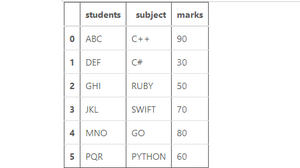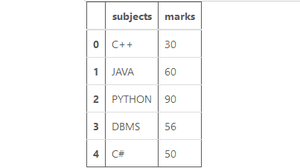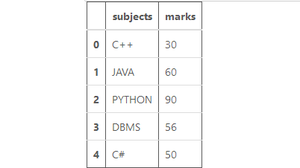# Combine two Pandas series into a DataFrame

In this post, we will learn how to combine two series into a DataFrame? Before starting let’s see what a series is?
Pandas Series is a one-dimensional labeled array capable of holding any data type. In other terms, Pandas Series is nothing but a column in an excel sheet.

There are several ways to concatenate two series in pandas. Following are some of the ways:

Method 1: Using pandas.concat().

This method does all of the heavy lifting of performing concatenation operations along an axis while performing optional set logic (union or intersection) of the indexes (if any) on the other axes.

Code:

## python

 `# import pandas library ` `import` `pandas as pd ` ` `  `# this user defines function ` `# creates a series ` `# from the passed list. ` `def` `createSeries (series_list): ` `   `  `  ``# create a series ` `  ``series_list ``=` `pd.Series(series_list) ` `   `  `  ``return` `series_list ` ` `  `# create a series of students ` `students ``=` `createSeries([``'ABC'``, ``'DEF'``, ` `                         ``'GHI'``, ``'JKL'``, ` `                         ``'MNO'``, ``'PQR'``])   ` `# create a series of subjects ` `subject ``=` `createSeries([``'C++'``, ``'C#'``,  ` `                        ``'RUBY'``, ``'SWIFT'``, ` `                        ``'GO'``, ``'PYTHON'``]) ` `# create a series of marks ` `marks ``=` `createSeries([``90``, ``30``,  ` `                      ``50``, ``70``,  ` `                      ``80``, ``60``]) ` `# create a dictonary ` `data ``=` `{``"students"``: students, ` `        ``"subject"``: subject, ` `        ``"marks"``: marks} ` ` `  `# Concatenating the series side ` `# by side as depicted by axis=1 ` `# If you want to concatenate the  ` `# series one below the other ` `# change the axis to zero. ` `df ``=` `pd.concat(data, ` `               ``axis ``=` `1``) ` ` `  `# show the dataframe ` `df`

Output:Method 2: Using Series.append().

This method is a shortcut to concat. This method concatenates along axis=0 i.e. rows. Series.append() can take multiple objects to concatenate.

Code:

## Python3

 `# import pandas library ` `import` `pandas as pd ` ` `  `# create a series ` `a ``=` `pd.Series([``"ABC"``, ``"DEF"``,  ` `               ``"GHI"``]) ` ` `  `# create a series ` `b ``=` `pd.Series([``"JKL"``, ``"MNO"``,  ` `               ``"PQR"``]) ` ` `  `# combine two series then ` `# create a dataframe ` `df ``=` `pd.DataFrame(a.append(b,  ` `                  ``ignore_index ``=` `True``)) ` `# show the dataframe ` `df`

Output:Method 3: Using pandas.merge().

Pandas have high performance in-memory join operations which is very similar to RDBMS like SQL. merge can be used for all database join operations  between dataframe or named series objects. You have to pass an extra parameter “name” to the series in this case.

Code:

## Python3

 `# import pandas library ` `import` `pandas as pd ` ` `  `# create a series ` `a ``=` `pd.Series([``"C++"``, ``"JAVA"``,  ` `               ``"PYTHON"``, ``"DBMS"``, ` `               ``"C#"``], name ``=` `"subjects"``) ` ` `  `# create a series ` `b ``=` `pd.Series([``"30"``, ``"60"``,  ` `               ``"90"``, ``"56"``,  ` `               ``"50"``], name ``=` `"marks"``) ` ` `  `# merge both series  ` `df ``=` `pd.merge(a, b, right_index ``=` `True``, ` `               ``left_index ``=` `True``) ` `# show the dataframe ` `df `

Output:Method 4: Using Dataframe.join().

This method can be used also to join two series but you have to convert one series into dataframe.

Code:

## Python3

 `# import pandas library ` `import` `pandas as pd ` ` `  `# create a series ` `a ``=` `pd.Series([``"C++"``, ``"JAVA"``,  ` `               ``"PYTHON"``, ``"DBMS"``,  ` `               ``"C#"``], name ``=` `"subjects"``) ` ` `  `# create a series ` `b ``=` `pd.Series([``"30"``, ``"60"``,  ` `               ``"90"``, ``"56"``,  ` `               ``"50"``], name ``=` `"marks"``) ` ` `  `# create a dataframe ` `a ``=` `pd.DataFrame(a) ` ` `  `# add series 'b'  ` `# into dataframe 'a' ` `df ``=` `a.join(b) ` ` `  `# show the dataframe ` `df `

Output:My Personal Notes arrow_drop_upCheck out this Author's contributed articles.

If you like GeeksforGeeks and would like to contribute, you can also write an article using contribute.geeksforgeeks.org or mail your article to contribute@geeksforgeeks.org. See your article appearing on the GeeksforGeeks main page and help other Geeks.

Please Improve this article if you find anything incorrect by clicking on the "Improve Article" button below.

Article Tags :

Be the First to upvote.

Please write to us at contribute@geeksforgeeks.org to report any issue with the above content.